# Nonchaotic Multidimensional Flows

## 9/19/00 Lecture #3 in Physics 505

###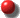Comments on Homework #1 (The Logistic Equation)

• Some people didn't note sensitive dependence on conditions
• Please write a short summary of your results for each question
• Course grades are available on the WWW (check for accuracy)

###Review (last week) - One Dimensional Maps (Logistic Map)

• Xn+1 = AXn(1 - Xn)
• Control parameters and bifurcations
• Stable and unstable fixed points (attractors and repellors)
• Basin of attraction
• Periodic cycles (periodic attractors and repellors)
• Period-doubling route to chaos
• Bifurcation diagram (self-similar fractal)
• Feigenbaum numbers ("Feigenvalues")
• Chaos and periodic windows
• Stretching and folding (sensitive dependence)
• Transient chaos
• Eventually fixed points (preimages)
• Cantor set (infinite set of measure zero)
• Eventually periodic points
• Dense unstable periodic orbits
• Period-3 implies chaos (Li and Yorke)
• Numerical errors in logistic map calculation
• Probability density: P = 1 / p[X(1 - X)]1/2
• Ergodic hypothesis
• Solution: Xn = (1 - cos(2ncos-1(1 - 2X0)))/2
• Can be used to make fractal music

###Maps versus Flows

 Maps: Flows: Discrete time Continuous time Variables change abruptly Variables change smoothly Described by algebraic equations Described by differential equations Complicated dynamics (in 1-D) Simple dynamics (in 1-D) Xn+1 = f(Xn) dx/dt = f(x) Example: Xn+1 = AXn Example: dx/dt = ax Solution: Xn = AnX0Solution: x = x0eat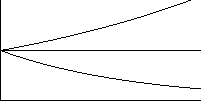Growth for A >1  Decay for A < 1 Growth for a > 0  Decay for a < 0 We call this an "orbit" We call this a "trajectory" n --> t,   A --> ea t --> n,   a --> loge A

###Logistic Differential Equation (1-D nonlinear flow)

• dx/dt = ax(1 - x[= f(x)]

• f(x)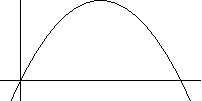x
• Used by Verhulst to model population growth (1838)
• Equilibria (fixed points):  f(x) = 0 ==> x* = 0, 1
• Stable if df/dx < 0, unstable if df/dx > 0 (evaluated at x*)
• All initial conditions in (0, 1) attract to x* = 1 for all a > 0
• Oscillations and chaos are impossible
• Only point attractors (and repellors) are possible
• There can be many equilibria in nonlinear systems

###Circular Motion (2-D linear flow)

• Ball on string, planetary motion, gyrating electron, etc.
• dx/dt = y,  dy/dt = -x, y0 = 0
• Solution:  x = x0 cos t  (or x0 sin t)
• Note:  x2 + y2 = x02 = constant (a circle of radius x0)
• Equilibrium point at (0, 0) is called a center

•• A center is neutrally stable (neither attracts nor repels)

###Mass on a Spring (frictionless)

• Newton's second lawF = ma  (a = dv/dt)
• Hooke's law (spring):  F = -kx
• Equation of motionmdv/dt = -kx
• This is a prototypical (linear) harmonic oscillator
• Let m = k = 1 (or define new variables)
• Then:  dv/dt = -xdx/dt = v
• Motion is a circle in phase space (x, v)
• 2 phase-space variables for each real variable
• This is because F = ma is a second order ODE
• Phase-space orbit is elliptical because energy is constant
• E = mv2 / 2 + kx2 / 2  ==>  x2 + v2 = constant  (for m = k = 1)
• There is no attractor for the motion (only a center)

###Nonautonomous Equations

• These have t on the right-hand side
• Example: driven mass on a spring
• md2x/dt2 = -kx + A sin wt
• Let m = k = 1 (for simplicity)
• dv/dt = -x + A sin wt
• dx/dt = v
• Let wt = f
• dx/dt = v
• dv/dt = -x + A sin f
• df/dt = w
• Can always eliminate t by adding a variable
• The f direction is periodic (period 2p)
• The motion is on a torus (a doughnut)

•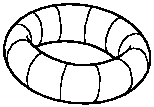###Damped Harmonic Oscillator

• Add friction to harmonic oscillator (i.e., F = -bdx/dt)
• dx/dt = v
• dv/dt = -x - bv
• Equilibriumx* = 0, v* = 0  (stable)
• Mechanical energy is not conserved (dissipation)
• Best undersood from phase portrait
• Types of equilibria for damped oscillator:
•  Spiral point (focus)  if b < 2 (underdamped) Radial point (node)  if b > 2 (overdamped)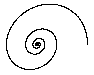• Attractor --> sink,  repellor --> source
• Trajectory cannot intersect itself
• Poincare-Bendixson theorem (no chaos in 2-D)
• Chaos in flows requires at least 3 variables and a nonlinearity (next time)

###Van der Pol Equation (2-D nonlinear ODE)

• Model for electrical oscillator, heart, Cephids
• dx/dt = y
• dy/dt = b(1 - x2)y - x
• Unstable equilibrium point (for b > 0):  x* = 0: y* = 0
• Growth for small x, ydecay for large x, y
• Solution attracts to a stable limit cycle

•• Basin of attraction is the whole x-y plane

###Numerical Methods for solving ODEs

• dx/dt = f(x, y),  dy/dt = g(x, y)
• Let h be a small interval of time (delta t)
• This converts to flow to a map
• Euler method:
• xn+1 = xn + hf(xn, yn)
•  yn+1 = yn + hg(xn, yn)
• x and y are advanced simultaneously
• This is a first-order method
• It works poorly (orbit spirals out)
• Leap-frog method:
• Good if f = f(y) and g = g(x)
• xn+2 = xn + hf( yn+1)
•  yn+3 = yn+1 + hg(xn+2)
• x and y are advanced sequentially
• This is a second-order method
• Periodic orbit orbit closes exactly
• Can be modified for f(xn, yn) and g(xn, yn)
• Second order Runge-Kutta method:
• Trial step: kx = hf(xn, yn), ky = hg(xn, yn)
• xn+1 = xn + hf(xn + kx/2, yn + ky/2)
•  yn+1 = yn + hg(xn + kx/2, yn + ky/2)
• Can be extended to higher order (see HW #3)
• Fourth order is a good compromise

J. C. Sprott | Physics 505 Home Page | Previous Lecture | Next Lecture